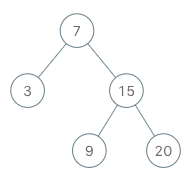# 173. Binary Search Tree Iterator (Medium)

https://leetcode.com/problems/binary-search-tree-iterator/

Implement an iterator over a binary search tree (BST). Your iterator will be initialized with the root node of a BST.

Calling `next()` will return the next smallest number in the BST.

Example:```BSTIterator iterator = new BSTIterator(root);
iterator.next();    // return 3
iterator.next();    // return 7
iterator.hasNext(); // return true
iterator.next();    // return 9
iterator.hasNext(); // return true
iterator.next();    // return 15
iterator.hasNext(); // return true
iterator.next();    // return 20
iterator.hasNext(); // return false
```

Note:

• `next()` and `hasNext()` should run in average O(1) time and uses O(h) memory, where h is the height of the tree.
• You may assume that `next()` call will always be valid, that is, there will be at least a next smallest number in the BST when `next()` is called.

## Solutions

https://www.programcreek.com/2014/04/leetcode-binary-search-tree-iterator-java/

``````class BSTIterator {
Stack<TreeNode> stack;

public BSTIterator(TreeNode root) {
stack = new Stack<>();

// left section is always smaller, visit left at first
pushLeft(root);
}

public boolean hasNext() {
return !stack.isEmpty();
}

public int next() {
TreeNode node = stack.pop();
int result = node.val;

// 'node' will be visited only if its left children nodes are all visited
// since 'node' visited, then visit its right children nodes
if (node.right != null) {
node = node.right;

pushLeft(node);
}

return result;
}

public void pushLeft(TreeNode node) {
while (node != null) {
stack.push(node);
node = node.left;
}
}
}
``````